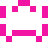### Real Vim ninjas count every keystroke - do you?

###### Pick a challenge, fire up Vim, and show us what you got.

```Your VimGolf key: please sign in

\$ gem install vimgolf
\$ vimgolf setup
\$ vimgolf put 59553bd164628d0009000038
```

### Missing Library and a Typo

##### Start file
```#define MIN_NUMBER 1
#define MAX_NUMBER 100
#include <stdio.h>
#include <time.h>
#include <stdlib.h>

int getGuess(){
int c;
int guess = 0;

while(guess < MIN_NUMBER || guess > MAX_NUMBER){
printf("Guess: ");
scanf("%d", &guess);

while((c = getchar()) != '\n' && c != EOF);
}

return guess;
}

int main(){
srand(time(NULL));
int number = rand()%(MAX_NUMBER-MIN_NUMBER+1)+MIN_NUMBER;

printf("Let's start a game.\n");
printf("I've picked a number between %d and %d\n",MIN_NUMBER,MAX_NUMBER);
printf("Guess what it is and I'll tell you \n");
printf("if it's higher or lower.\n");
printf("You win if you guess it in 7 turns or less\n\n");

int turn;
for(turn = 0; turn < 7; turn++){
int guess = getGuess();
assert(guess>=MIN_NUMBER && guess<=MAX_NUMBER);

if(guess < number){
printf("Higher\n\n");
}else if(guess > number){
printf("Lower\n\n");
}else{
break;
}
}

if(turn < 7)
printf("\nYou Win!\n");
else
printf("You Lose the answer was %d.\n", number);

return 0;
}
// I think I forgot to include the library assetr
```
##### End file
```#define MIN_NUMBER 1
#define MAX_NUMBER 100
#include <stdio.h>
#include <time.h>
#include <stdlib.h>
#include <assert.h>

int getGuess(){
int c;
int guess = 0;

while(guess < MIN_NUMBER || guess > MAX_NUMBER){
printf("Guess: ");
scanf("%d", &guess);

while((c = getchar()) != '\n' && c != EOF);
}

return guess;
}

int main(){
srand(time(NULL));
int number = rand()%(MAX_NUMBER-MIN_NUMBER+1)+MIN_NUMBER;

printf("Let's start a game.\n");
printf("I've picked a number between %d and %d\n",MIN_NUMBER,MAX_NUMBER);
printf("Guess what it is and I'll tell you \n");
printf("if it's higher or lower.\n");
printf("You win if you guess it in 7 turns or less\n\n");

int turn;
for(turn = 0; turn < 7; turn++){
int guess = getGuess();
assert(guess>=MIN_NUMBER && guess<=MAX_NUMBER);

if(guess < number){
printf("Higher\n\n");
}else if(guess > number){
printf("Lower\n\n");
}else{
break;
}
}

if(turn < 7)
printf("\nYou Win!\n");
else
printf("You Lose the answer was %d.\n", number);

return 0;
}
// I think I forgot to include the library assert
```

#### View Diff

```5a6
> #include <assert.h>
52c53
< // I think I forgot to include the library assetr
---
> // I think I forgot to include the library assert
```

### Solutions by @songz:

Unlock 3 remaining solutions by signing in and submitting your own entry

## 207 active golfers, 840 entries

##### Solutions by @songz:16
###### #26 - Song Zheng / @songz

06/29/2017 at 09:14PM17
###### #>102 - Song Zheng / @songz

06/29/2017 at 09:08PM18
###### #>118 - Song Zheng / @songz

06/29/2017 at 08:59PM